SSC BOARD PAPERS IMPORTANT TOPICS COVERED FOR BOARD EXAM 2024

### Question 12. Solve the following example. An object thrown vertically upwards reaches a height of 500 m. What was its initial velocity? How long will the object take to come back to the earth? Assume g = 10 m/s2

Question 12.

Solve the following example.

An object thrown vertically upwards reaches a height of 500 m. What was its initial velocity? How long will the object take to come back to the earth? Assume g = 10 m/s2

From newton’s third equation of the motion;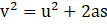Where;

V = Final velocity;

U = initial velocity;

T = time taken;

S = distance travelled;

A = acceleration;

According to our question;

The figure below illustrates the situation given in the question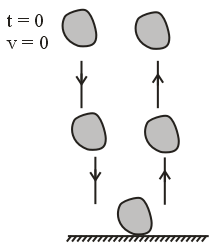V = 0 (Velocity at maximum height is zero);

S = 500m;

A = -10m/s2 (because when object will be going up the acceleration due to gravity will be acting downwards to make object to fall. Hence by sign convention direction of motion and acceleration is opposite therefore a is negative);

Putting the above values we get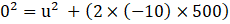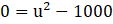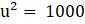⇒u= √1000=100

Therefore initial velocity is 100m/s.

From the Newton’s first law of motion;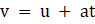Where symbols have there usual meanings as above;

v = 0 (velocity at maximum height is zero);

u = initial velocity = 100m/s;

a = - 10m/s2 (because when object will be going up the acceleration due to gravity will be acting downwards to make object to fall. Hence by sign convention direction of motion and acceleration is opposite therefore a is negative);

Putting the values we get;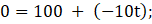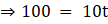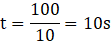Now we know that time required by an object to go up is same as time required to come down.

Therefore;

Total time = time of ascent + time of descent = 10 + 10 = 20 s

Hence total time to come back to earth is 20 seconds.